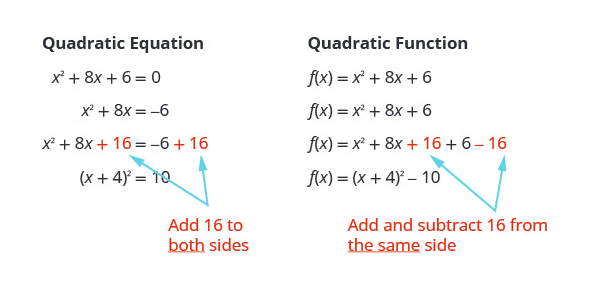Quadratic Functions And Equations Quiz 1

21 Questions | Total Attempts: 2912SettingsThere are different ways that one can use to solve a quadratic function and equations, which were covered in Lessons 8-1, 8-2, 8-3, and 8-6. If you have just learnt how to solve these equations then the practice quiz below is perfect for you. Give it a shot and get to sharpen your skills.

• 1.
The vertex of this parabola shows that the __________ value of the function is _____.
• A.

Minimum; 2

• B.

Maximum; 4

• C.

Minimum; 4

• D.

Maximum; 2

• 2.
Which of the following statements is always true?
• A.

The graph of a quadratic function is a straight line.

• B.

The range of a quadratic function is the set of all real numbers.

• C.

The highest power of the independent variable in a quadratic function is 2.

• D.

The vertex of a parabola occurs at the minimum value of the function.

• 3.
Which of the following statements is never true?
• A.

The vertex of a parabola occurs in the first quadrant.

• B.

The graph of a quadratic function that has a maximum opens upward.

• C.

The graph of a quadratic function contains the point (0, 0).

• D.

The axis of symmetry passes through the vertex.

• 4.
The vertex of this parabola shows that the __________ value of the function is _____.
• A.

Minimum; -5

• B.

Maximum; 1

• C.

Minimum; -9

• D.

Maximum; 2

• 5.
Find the axis of symmetry of the parabola with zeros -1 and 6
• A.

X = 1.5

• B.

X = 2

• C.

X = 2.5

• D.

X = 3

• 6.
The vertex of a quadratic function is in the second quadrant.  The related equation has no real solutions.  Which of the following statements is true?
• A.

The graph opens downward.

• B.

The graph opens upward.

• C.

The y-intercept is 0.

• D.

The axis of symmetry is x = 0.

Related TopicsBack to top